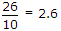# Electronics and Communication Engineering - Microprocessors

### Exercise :: Microprocessors - Section 1

26.

In a microprocessor

 A. all instructions need only one machine cycle B. some instructions need only one machine cycle while some other instructions need more than one machine cycle C. all instructions need only fraction of one machine cycle D. some instructions need less than one machine cycle

Explanation:

Some instructrions do need more than one machine cycle.

27.

Assertion (A): If source and destination addresses are made implicit the length of instruction is reduced.

Reason (R): 8085 has only 3 addressing modes.

 A. Both A and R are correct and R is correct explanation of A B. Both A and R are correct but R is not correct explanation of A C. A is correct R is wrong D. A is wrong R is correct

Explanation:

The number of addressing modes is five.

28.

Assertion (A): Microprocessor 8085 has on-chip oscillator with inbuilt crystal.

Reason (R): For frequency stability crystal oscillator is preferred.

 A. Both A and R are correct and R is correct explanation of A B. Both A and R are correct but R is not correct explanation of A C. A is correct R is wrong D. A is wrong R is correct

Explanation:

Crystal has to be connected externally to pins 1 and 2 of 8085.

29.

Consider the following program in Pascal `X = 2.57X = (X + 0.06) * 10I = TRUNC (X)X = IX = X / 10.0` The final value of X would be

 A. 2 B. 2.6 C. 2.63 D. 26.3

Explanation:

(2.57 + 0.06) 10 = 26.3 →.

30.

Consider the following

1. The first letter of a Java variable is lower case.
2. Each successive word in Java variable begins with a capital letter.
3. All other letters are lower case.
4. A Java variable can have a digit as first character.
Which of the above are correct?

 A. All B. 1, 2, 3 only C. 1, 2, 4 only D. 2, 3, 4 only